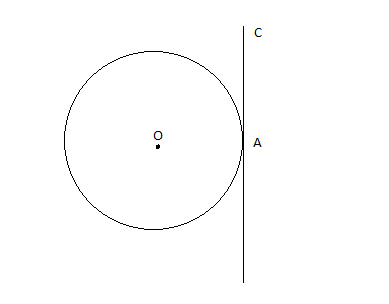Filters
Latest Questions
Mathematics
Tangents and normals of a circle
Find the equation of the circle which touches the line $4x – 3y + 10 = 0$ and $4x – 3y – 30 = 0$ and whose centre lies on the line $2x + y = 0$

Mathematics
Tangents and normals of a circle
If $a > 2b > 0$, then the positive value of m for which $y = mx - b\sqrt {1 + {m^2}}$ is a common tangent to ${x^2} + {y^2} = {b^2}$ and ${\left( {x - a} \right)^2} + {y^2} = {b^2}$ is:
A. $\dfrac{{2b}}{{\sqrt {{a^2} - 4{b^2}} }}$
B. $\dfrac{{\sqrt {{a^2} - 4{b^2}} }}{{2b}}$
C. $\dfrac{{2b}}{{a - 2b}}$
D. $\dfrac{b}{{a - 2b}}$
Mathematics
Tangents and normals of a circle
What is the Point of tangency in a circle?
Mathematics
Tangents and normals of a circle
The equation of the tangent to the circle ${{x}^{2}}+{{y}^{2}}={{a}^{2}}$ , which makes a triangle of area ${{a}^{2}}$ with the coordinate axes, is
A. $x\pm y=\pm a$
B. $x\pm y=\pm a\sqrt{2}$
C. $x\pm y=3a$
D. $x\pm y=\pm 2a$
Mathematics
Tangents and normals of a circle
Draw a circle of radius 4 cm. Construct a pair of tangents to it, the angle between which is 60°. Also justify the construction. Measure the distance between the centre of the circle and the point of intersection of tangents.
A. 4 cm
B. 6 cm
C. 8 cm
D. 10 cm
Mathematics
Tangents and normals of a circle
Show that the circles touch each other externally. Find the point of contact and equation of their common tangent.
${x^2} + {y^2} - 4x + 10y + 20 = 0$
${x^2} + {y^2} + 8x - 6y - 24 = 0$
Mathematics
Tangents and normals of a circle
Find the point of contact in a circle.Mathematics
Tangents and normals of a circle
If from any point $P,$ tangents $PT,P{T}'$ are drawn to two given circles with centers $A$ and $B$ respectively; and if $PN$ is the perpendicular from $P$ on their radical axis, then $P{{T}^{2}}-P{{{T}'}^{2}}=$
$\left( a \right) 2.PN.AB$
$\left( b \right) 4.PN.AB$
$\left( c \right) PN.AB$
$\left( d \right)$ None of these
Mathematics
Tangents and normals of a circle
How do you find the gradient of the tangent to the curve $y = {x^3}$ at the given value of $x = 4$ ?
Mathematics
Tangents and normals of a circle
The center of the circle(s) passing through the points (0, 0), (1, 0) and touching the circle ${{x}^{2}}+{{y}^{2}}=16$, is(are)
(a) $\left( \dfrac{1}{2},\dfrac{\sqrt{15}}{2} \right)$
(b) $\left( \dfrac{1}{2},-\dfrac{\sqrt{15}}{2} \right)$
(c) $\left( \dfrac{1}{2},{{2}^{\dfrac{1}{2}}} \right)$
(d) $\left( \dfrac{1}{2},-{{2}^{\dfrac{1}{2}}} \right)$
Mathematics
Tangents and normals of a circle
Assertion
Tangents are drawn from the point (17,7) to the circle ${x^2} + {y^2} = 169$ . Statement-1 The tangents are mutually perpendicular:
Reason
Statement-2: The locus of the points from which mutually perpendicular tangents can be drawn to the given circle is ${x^2} + {y^2} = 338$
A Both Assertion and Reason are correct and Reason is the correct explanation for Assertion
B Both Assertion and Reason are correct but Reason is not the correct explanation for Assertion
C Assertion is correct but Reason is incorrect
D Assertion is incorrect but Reason are correct

Mathematics
Tangents and normals of a circle
If.${v_1},{v_2},{v_3}$. are the volumes of parallelepiped, triangular prism and tetrahedron respectively and three conterminous edges of all figures are $\mathop a\limits^ \to ,\mathop b\limits^ \to ,\mathop c\limits^ \to$ then ${v_1},{v_2},{v_3}$ equals to
$A)\,1:3:6 \\ B)\,6:1:3 \\ C)\,6:3:1 \\ D)None\,\,of\,these \\$
Prev
1
2
3
4